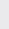Course Content

0/4

0/3

0/1

0/2

0/1

0/4

0/3

0/5

0/3

0/5

0/5

0/1

0/6

0/2

0/4

0/5

11.5 Dividing Ratio Formula 分比公式

1. A(2, -3), B(h, k) and C(-3, 4) three points are located at the same straight line such that 5AB = 2BC. Find the values of h and k.

A(2, -3), B(h, k) 和 C(-3, 4) 三点在一条线上的点且5AB = 2BC. 求 h 和 k的., –1
2. P(5, p) separate the segment connecting A(3, 7) and B(6, 2) with ratio m : n. Find
P(5, p) 把连接 A(3, 7) 和 B(6, 2) 的线段分成比例 m : n. 求

(a) the ratio m : n,

(b) the value of p.
p 的值。

(a) 2 : 1

(b) 33. Segment PQ is divided by point R externally such that RP =RQ. If coordinates of Q and R are (8, -3) and (-16, 9) respectively, find the coordinate of P.

PQ 线段被点 R 外分使得 RP =RQ. 若 Q 和 R 的坐标分别是 (8, -3) 和 (-16, 9), 求 P点的坐标.

(–4, 3)
4. Points A(14, 6) and B(5, 11), given that the area ofOAP is twice of the area ofOPB where O is the origin and point P lies between A and B. Find the coordinate of point P.

(8, 9)
5. Given the points A(-2, 1), B(3, 6). Find the point M which divides line AB in the ratio AM : MB = 3 : 2.

(1, 4)
6. On a straight line A(-2,1) and B(2,7), find the coordinates of P if

(a) P divide AB into 3AP = 2PB internally

P內分直线AB成3AP = 2PB,

(b) P divide AB into 5AP = – AB externally

P外分直线AB成5AP = – AB,

(a)

*** QuickLaTeX cannot compile formula:
\left(-\frac{2}{5}

*** Error message:
Missing \right. inserted.
leading text: $\left(-\frac{2}{5}$



,

*** QuickLaTeX cannot compile formula:
\ 3\frac{2}{5})\right

*** Error message:
Missing delimiter (. inserted).
leading text: $\ 3\frac{2}{5})\right$



(b)

*** QuickLaTeX cannot compile formula:
\left(-2\frac{4}{5}

*** Error message:
Missing \right. inserted.
leading text: $\left(-2\frac{4}{5}$



,

*** QuickLaTeX cannot compile formula:
\ -\frac{1}{5})\right

*** Error message:
Missing delimiter (. inserted).
leading text: $\ -\frac{1}{5})\right$



7.ABC with vertices A(8, 10), B(0, -10) and C(12, -2), P and Q lie on AB and AC respectively. If PQ // BC, and the ratio of the area ofAPQ to the area ofABC is 1 : 16, calculate the coordinates of the midpoint of PQ.ABC之顶点A(8, 10), B(0, -10) 和C(12, -2), P 和Q 分別在AB 和AC上. 若PQ // BC, 且APQ 和ABC 的面积比为1 : 16, 求PQ的中点座标.

(7, 6)
8. InABC, A(1, 2), B(9, 18) and C(13, -6). If P divide AB into 3 : 1 internally, Q on the segment AC andAPQ :ABC = 1 : 2, find the coordinates of P, Q.ABC之顶点为A(1, 2), B(9, 18) 及C(13, -6). 若P內分AB之比为3 : 1, Q为线段AC上一点, 且APQ :ABC＝1 : 2, 求P, Q之座标。
(7, 14), (9, -3)